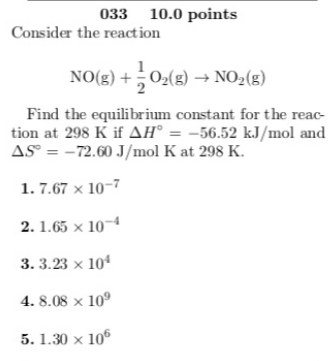# Consider the reaction NO(g) + 1/2O2(g) → NO2(g) Find the equilibrium constant for the reaction at 298 K if ΔHdegrees = -56.52 kJ/mol and ΔS degrees = -72.60 J/mol K at 298 K. 1. 1.67 x 10^-7 2. 1.65 x 10^-4 3. 3.23 x 10^4 4. 8.08 x 10^9 5. 1.30 x 10^6